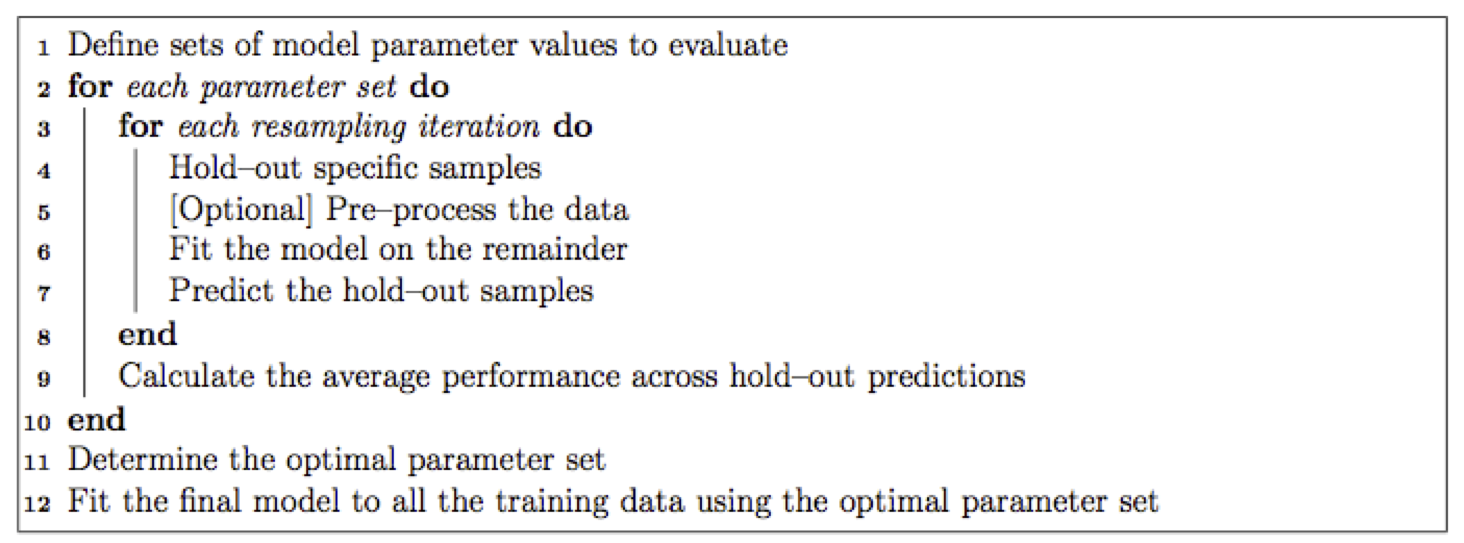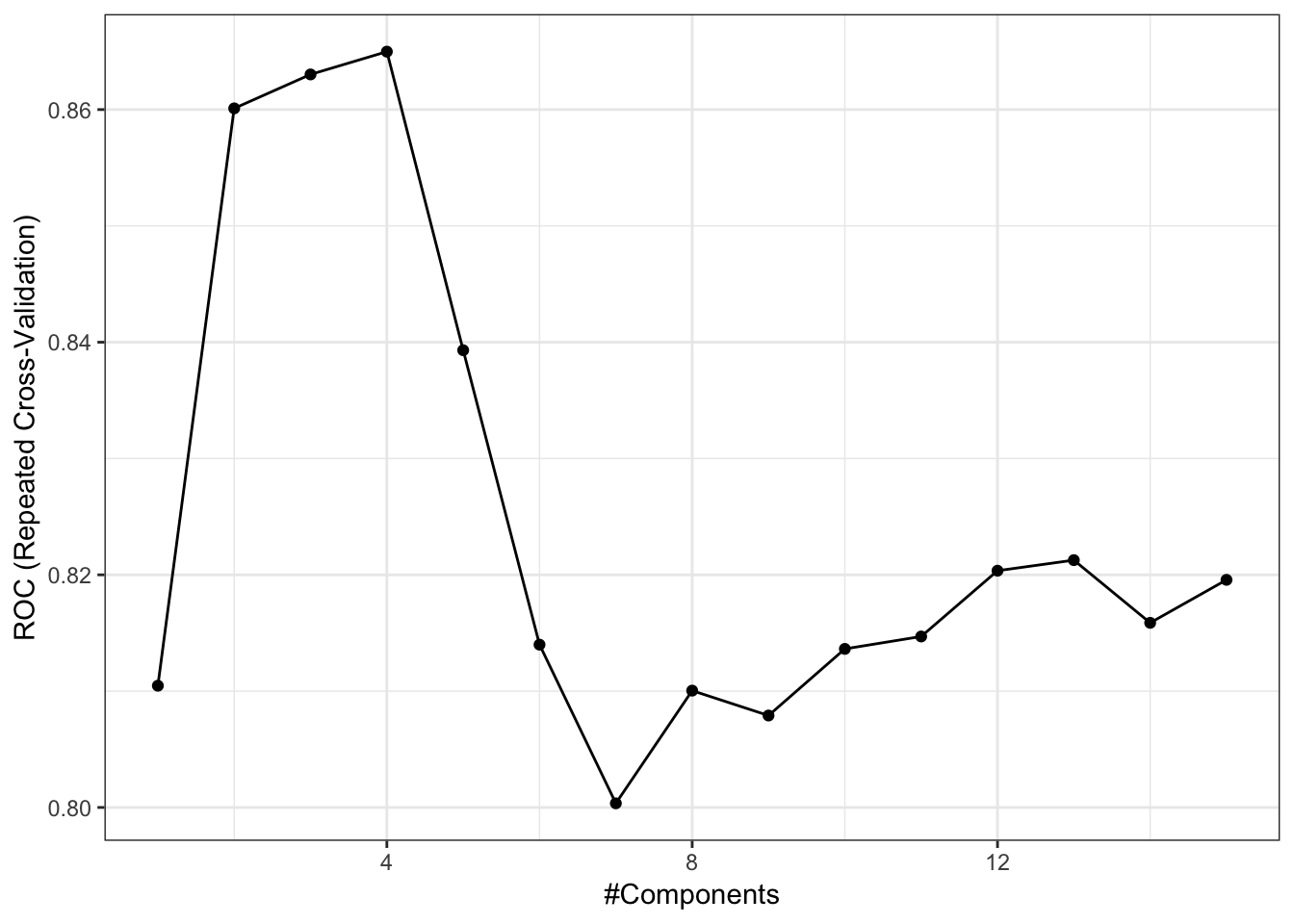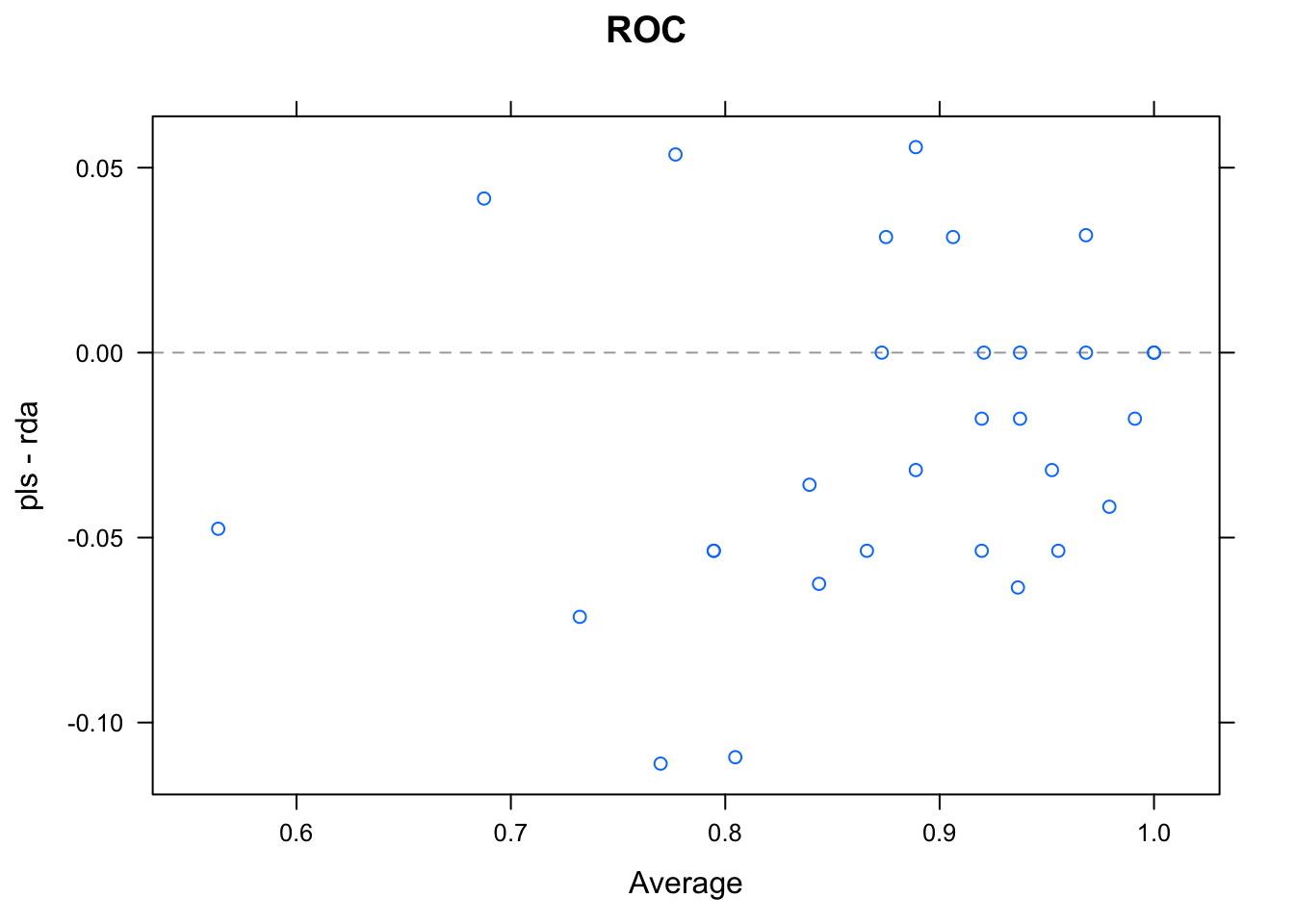# A Short Introduction to the caret Package

The caret package (short for Classification And REgression Training) contains functions to streamline the model training process for complex regression and classification problems. The package utilizes a number of R packages but tries not to load them all at package start-up (by removing formal package dependencies, the package startup time can be greatly decreased). The package “suggests” field includes 30 packages. caret loads packages as needed and assumes that they are installed. If a modeling package is missing, there is a prompt to install it.

Install caret using

install.packages("caret", dependencies = c("Depends", "Suggests"))

to ensure that all the needed packages are installed.

The main help pages for the package are at https://topepo.github.io/caret/ Here, there are extended examples and a large amount of information that previously found in the package vignettes.

caret has several functions that attempt to streamline the model building and evaluation process, as well as feature selection and other techniques.

One of the primary tools in the package is the train function which can be used to

• evaluate, using resampling, the effect of model tuning parameters on performance
• choose the optimal’’ model across these parameters
• estimate model performance from a training set

More formally:There are options for customizing almost every step of this process (e.g. resampling technique, choosing the optimal parameters etc). To demonstrate this function, the Sonar data from the mlbench package will be used.

The Sonar data consist of 208 data points collected on 60 predictors. The goal is to predict the two classes M for metal cylinder or R for rock).

First, we split the data into two groups: a training set and a test set. To do this, the createDataPartition function is used:

library(caret)
library(mlbench)
data(Sonar)

set.seed(107)
inTrain <- createDataPartition(
y = Sonar$Class, ## the outcome data are needed p = .75, ## The percentage of data in the ## training set list = FALSE ) ## The format of the results ## The output is a set of integers for the rows of Sonar ## that belong in the training set. str(inTrain) #> int [1:157, 1] 1 2 3 6 7 9 10 11 12 13 ... #> - attr(*, "dimnames")=List of 2 #> ..$ : NULL
#>   ..$: chr "Resample1" By default, createDataPartition does a stratified random split of the data. To partition the data: training <- Sonar[ inTrain,] testing <- Sonar[-inTrain,] nrow(training) #>  157 nrow(testing) #>  51 To tune a model using the algorithm above, the train function can be used. More details on this function can be found at https://topepo.github.io/caret/model-training-and-tuning.html. Here, a partial least squares discriminant analysis (PLSDA) model will be tuned over the number of PLS components that should be retained. The most basic syntax to do this is: plsFit <- train( Class ~ ., data = training, method = "pls", ## Center and scale the predictors for the training ## set and all future samples. preProc = c("center", "scale") ) However, we would probably like to customize it in a few ways: • expand the set of PLS models that the function evaluates. By default, the function will tune over three values of each tuning parameter. • the type of resampling used. The simple bootstrap is used by default. We will have the function use three repeats of 10-fold cross-validation. • the methods for measuring performance. If unspecified, overall accuracy and the Kappa statistic are computed. For regression models, root mean squared error and R2 are computed. Here, the function will be altered to estimate the area under the ROC curve, the sensitivity and specificity To change the candidate values of the tuning parameter, either of the tuneLength or tuneGrid arguments can be used. The train function can generate a candidate set of parameter values and the tuneLength argument controls how many are evaluated. In the case of PLS, the function uses a sequence of integers from 1 to tuneLength. If we want to evaluate all integers between 1 and 15, setting tuneLength = 15 would achieve this. The tuneGrid argument is used when specific values are desired. A data frame is used where each row is a tuning parameter setting and each column is a tuning parameter. An example is used below to illustrate this. plsFit <- train( Class ~ ., data = training, method = "pls", preProc = c("center", "scale"), ## added: tuneLength = 15 ) To modify the resampling method, a trainControl function is used. The option method controls the type of resampling and defaults to "boot". Another method, "repeatedcv", is used to specify repeated K-fold cross-validation (and the argument repeats controls the number of repetitions). K is controlled by the number argument and defaults to 10. The new syntax is then: ctrl <- trainControl(method = "repeatedcv", repeats = 3) plsFit <- train( Class ~ ., data = training, method = "pls", preProc = c("center", "scale"), tuneLength = 15, ## added: trControl = ctrl ) Finally, to choose different measures of performance, additional arguments are given to trainControl. The summaryFunction argument is used to pass in a function that takes the observed and predicted values and estimate some measure of performance. Two such functions are already included in the package: defaultSummary and twoClassSummary. The latter will compute measures specific to two-class problems, such as the area under the ROC curve, the sensitivity and specificity. Since the ROC curve is based on the predicted class probabilities (which are not computed automatically), another option is required. The classProbs = TRUE option is used to include these calculations. Lastly, the function will pick the tuning parameters associated with the best results. Since we are using custom performance measures, the criterion that should be optimized must also be specified. In the call to train, we can use metric = "ROC" to do this. ctrl <- trainControl( method = "repeatedcv", repeats = 3, classProbs = TRUE, summaryFunction = twoClassSummary ) set.seed(123) plsFit <- train( Class ~ ., data = training, method = "pls", preProc = c("center", "scale"), tuneLength = 15, trControl = ctrl, metric = "ROC" ) plsFit #> Partial Least Squares #> #> 157 samples #> 60 predictor #> 2 classes: 'M', 'R' #> #> Pre-processing: centered (60), scaled (60) #> Resampling: Cross-Validated (10 fold, repeated 3 times) #> Summary of sample sizes: 141, 141, 142, 141, 142, 141, ... #> Resampling results across tuning parameters: #> #> ncomp ROC Sens Spec #> 1 0.810 0.716 0.730 #> 2 0.860 0.770 0.800 #> 3 0.863 0.762 0.820 #> 4 0.865 0.766 0.782 #> 5 0.839 0.728 0.774 #> 6 0.814 0.752 0.788 #> 7 0.800 0.708 0.759 #> 8 0.810 0.707 0.759 #> 9 0.808 0.715 0.764 #> 10 0.814 0.712 0.772 #> 11 0.815 0.712 0.759 #> 12 0.820 0.724 0.762 #> 13 0.821 0.733 0.758 #> 14 0.816 0.741 0.758 #> 15 0.820 0.725 0.749 #> #> ROC was used to select the optimal model using #> the largest value. #> The final value used for the model was ncomp = 4. In this output the grid of results are the average resampled estimates of performance. The note at the bottom tells the user that 4 PLS components were found to be optimal. Based on this value, a final PLS model is fit to the whole data set using this specification and this is the model that is used to predict future samples. The package has several functions for visualizing the results. One method for doing this is the ggplot function for train objects. The command ggplot(plsFit) produced the results seen in Figure and shows the relationship between the resampled performance values and the number of PLS components. ggplot(plsFit)To predict new samples, predict.train can be used. For classification models, the default behavior is to calculate the predicted class. The option type = "prob" can be used to compute class probabilities from the model. For example: plsClasses <- predict(plsFit, newdata = testing) str(plsClasses) #> Factor w/ 2 levels "M","R": 2 1 1 2 1 2 2 2 2 2 ... plsProbs <- predict(plsFit, newdata = testing, type = "prob") head(plsProbs) #> M R #> 4 0.441 0.559 #> 5 0.545 0.455 #> 8 0.631 0.369 #> 16 0.348 0.652 #> 20 0.783 0.217 #> 25 0.249 0.751 caret contains a function to compute the confusion matrix and associated statistics for the model fit: confusionMatrix(data = plsClasses, testing$Class)
#> Confusion Matrix and Statistics
#>
#>           Reference
#> Prediction  M  R
#>          M 17  6
#>          R 10 18
#>
#>                Accuracy : 0.686
#>                  95% CI : (0.541, 0.809)
#>     No Information Rate : 0.529
#>     P-Value [Acc > NIR] : 0.0167
#>
#>                   Kappa : 0.376
#>
#>  Mcnemar's Test P-Value : 0.4533
#>
#>             Sensitivity : 0.630
#>             Specificity : 0.750
#>          Pos Pred Value : 0.739
#>          Neg Pred Value : 0.643
#>              Prevalence : 0.529
#>          Detection Rate : 0.333
#>    Detection Prevalence : 0.451
#>       Balanced Accuracy : 0.690
#>
#>        'Positive' Class : M
#> 

To fit an another model to the data, train can be invoked with minimal changes. Lists of models available can be found at https://topepo.github.io/caret/available-models.html or https://topepo.github.io/caret/train-models-by-tag.html. For example, to fit a regularized discriminant model to these data, the following syntax can be used:

## To illustrate, a custom grid is used
rdaGrid = data.frame(gamma = (0:4)/4, lambda = 3/4)
set.seed(123)
rdaFit <- train(
Class ~ .,
data = training,
method = "rda",
tuneGrid = rdaGrid,
trControl = ctrl,
metric = "ROC"
)
rdaFit
#> Regularized Discriminant Analysis
#>
#> 157 samples
#>  60 predictor
#>   2 classes: 'M', 'R'
#>
#> No pre-processing
#> Resampling: Cross-Validated (10 fold, repeated 3 times)
#> Summary of sample sizes: 141, 141, 142, 141, 142, 141, ...
#> Resampling results across tuning parameters:
#>
#>   gamma  ROC    Sens   Spec
#>   0.00   0.826  0.773  0.811
#>   0.25   0.888  0.788  0.800
#>   0.50   0.881  0.781  0.782
#>   0.75   0.871  0.761  0.737
#>   1.00   0.756  0.702  0.661
#>
#> Tuning parameter 'lambda' was held constant at a
#>  value of 0.75
#> ROC was used to select the optimal model using
#>  the largest value.
#> The final values used for the model were gamma =
#>  0.25 and lambda = 0.75.
rdaClasses <- predict(rdaFit, newdata = testing)
confusionMatrix(rdaClasses, testing\$Class)
#> Confusion Matrix and Statistics
#>
#>           Reference
#> Prediction  M  R
#>          M 22  5
#>          R  5 19
#>
#>                Accuracy : 0.804
#>                  95% CI : (0.669, 0.902)
#>     No Information Rate : 0.529
#>     P-Value [Acc > NIR] : 4.34e-05
#>
#>                   Kappa : 0.606
#>
#>  Mcnemar's Test P-Value : 1
#>
#>             Sensitivity : 0.815
#>             Specificity : 0.792
#>          Pos Pred Value : 0.815
#>          Neg Pred Value : 0.792
#>              Prevalence : 0.529
#>          Detection Rate : 0.431
#>    Detection Prevalence : 0.529
#>       Balanced Accuracy : 0.803
#>
#>        'Positive' Class : M
#> 

How do these models compare in terms of their resampling results? The resamples function can be used to collect, summarize and contrast the resampling results. Since the random number seeds were initialized to the same value prior to calling train}, the same folds were used for each model. To assemble them:

resamps <- resamples(list(pls = plsFit, rda = rdaFit))
summary(resamps)
#>
#> Call:
#> summary.resamples(object = resamps)
#>
#> Models: pls, rda
#> Number of resamples: 30
#>
#> ROC
#>      Min. 1st Qu. Median  Mean 3rd Qu. Max. NA's
#> pls 0.540   0.806  0.899 0.865   0.935    1    0
#> rda 0.587   0.858  0.899 0.888   0.964    1    0
#>
#> Sens
#>      Min. 1st Qu. Median  Mean 3rd Qu. Max. NA's
#> pls 0.375   0.667  0.764 0.766   0.885    1    0
#> rda 0.375   0.688  0.826 0.788   0.885    1    0
#>
#> Spec
#>      Min. 1st Qu. Median  Mean 3rd Qu. Max. NA's
#> pls 0.286   0.714   0.75 0.782   0.857    1    0
#> rda 0.429   0.714   0.75 0.800   0.969    1    0

There are several functions to visualize these results. For example, a Bland-Altman type plot can be created using

xyplot(resamps, what = "BlandAltman")The results look similar. Since, for each resample, there are paired results a paired t-test can be used to assess whether there is a difference in the average resampled area under the ROC curve. The diff.resamples function can be used to compute this:

diffs <- diff(resamps)
summary(diffs)
#>
#> Call:
#> summary.diff.resamples(object = diffs)
#>
#> p-value adjustment: bonferroni
#> Upper diagonal: estimates of the difference
#> Lower diagonal: p-value for H0: difference = 0
#>
#> ROC
#>     pls     rda
#> pls         -0.0228
#> rda 0.00776
#>
#> Sens
#>     pls   rda
#> pls       -0.0227
#> rda 0.236
#>
#> Spec
#>     pls   rda
#> pls       -0.0179
#> rda 0.423`

Based on this analysis, the difference between the models is -0.023 ROC units (the RDA model is slightly higher) and the two-sided p-value for this difference is 0.008.